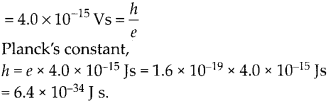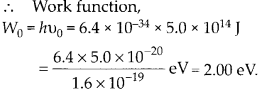Enlightened

# Question 28: NCERT Solutions for 12th Class Physics: Chapter 11-Dual Nature of Radiation and Matter

• 0

Question 28: NCERT Solutions for 12th Class Physics: Chapter 11-Dual Nature of Radiation and Matter

A mercury lamp is a convenient source for studying frequency dependence of photoelectric emission, since it gives a number of spectral lines ranging from the UV to the red end of the visible spectrum. In our experiment with rubidium photo-cell, the following lines from a mercury source were used :The stopping voltages, respectively, were measured to be:Determine the value of Planck’s constant h, the threshold frequency and work function for the material.

Share

1. Solution:
In order to calculate Planck’s constant ‘ll’ we need slope of the graph between cut off voltage and frequency. So, let us first calculate the frequency (u = c/λ) in each case and the table shows corresponding stopping potential.

V0 versus n plot shows that the first four points lie nearly on a straight line which intercepts the x-axis at threshold frequency, u0 = 5.0 × 1014 Hz. The fifth point u (= 4.3 × 1014 Hz) corresponds to u < u0, so there is no photoelectric emission and no stopping voltage is required to stop the current.
Slope of, V0 versus u graph is(b) threshold frequency, v0 = 5.0 × 1014 JCheck the complete chapter with solutions.
NCERT Solutions for 12th Class Physics: Chapter 11-Dual Nature of Radiation and Matter

• 0